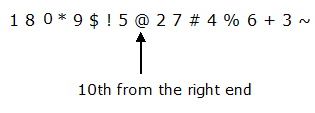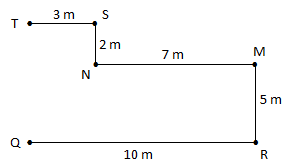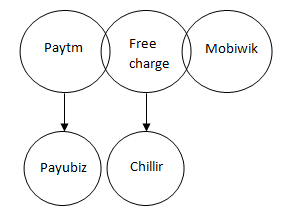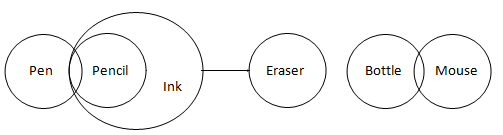# REASONING MISCELLANEOUS – 4

1 – 5) Study the following digit-letter-symbol sequence carefully and answer the given questions below.

1 G 8 V 0 U T * B 9 \$ H X ! 5 @ E 2 7 # I 4 S Y % 6 M J + A 3 Z ~ O

1) Which of the following is seventh to the right of thirteenth from the left end of the above arrangement?

a) E

b) I

c) 2

d) 7

e) #

e) #

1 G 8 V 0 U T * B 9 \$ H X ! 5 @ E 2 7 # I 4 S Y % 6 M J + A 3 Z ~ O

2) How many such vowels are there in the above arrangement each of which is immediately preceded by a symbol and immediately followed by a number?

a) None

b) One

c) Two

d) Three

e) None of these

d) Three

@ E 2

# I 4

+ A 3

3) Which of the following is sixteenth to the left of the seventh from the right of 6?

a) E

b) @

c) 2

d) 7

e) None of these

a) E

Sixteenth from the left of the seventh from the right of 6 => E

4) How many such numbers are there in the above arrangement each of which is immediately followed by a consonant?

a) One

b) Two

c) Four

d) Three

e) None of these

c) Four

8 V

4 S

3 Z

6 M

5) If all the letters are dropped from the above arrangement, which of the following will be the tenth from the right end?

a) 5

b) @

c) 7

d) 9

e) None of these

b) @6) Point R is 10m east of point Q. Point M is 5m north of Point R. Point M is 7m east of point N. Point N is 2m south of point S. Point T is 3m west of Point S. What is the distance between Point T and Point Q?

a) 5m

b) 8m

c) 7m

d) 10m

e) 13m

c) 7m7) Among five friends Kevin, Ramesh, Ravi, Rohit and Raju, Kevin is taller than Ramesh but shorter than Ravi and Ramesh is shorter than Rohit, who is the second tallest. Ravi is not the tallest, and then Ramesh is?

a) Tallest

b) Second shortest

c) Third tallest

d) Shortest

d) Shortest

Raju > Rohit > Ravi > Kevin > Ramesh

D.8 – 9) In each of the following questions some statements are given and these statements are followed by four conclusions numbered (I), (II), (III) and (IV). You have to take the given statements to be true even if they seem to be at variance from commonly known facts. Read the conclusions and then decide which of the given conclusions logically follows from the given statements.

10) Statements:

Some Paytm is Freecharge.

Some Freecharge is Mobiwik.

No Freecharge is Chillir.

No Payubiz is Paytm.

Conclusions:

I. Some Paytm being Mobiwik is a possibility.

II. Many Chillir are not Paytm.

III. Some Payubiz is Freecharge.

IV. No Payubiz is Freecharge.

a) Only I follows.

b) Only II and I follow.

c) Only IV and II follow.

d) Only IV follows.

e) None of these.

e) None of theseI. True

II. False

III. False

IV. False

Only I and either III or IV follows

8) Statements:

Some A is B.

Some B is C.

No D is C.

No E is D.

Conclusions:

I. All B is C.

II. No E is C.

III. Some A being E is a possibility.

IV. Some C is E.

a) Either II or IV follows.

b) Either II or IV and I follow.

c) Only III follows.

d) All follows.

e) Either II or IV and III follow.

E.Either II or IV and III Follow

9) Statements:

Some Pen are Pencil

No Eraser is Ink

All Pencil are Ink

Some Bottle are Mouse

Conclusions:

I. Some Mouse are Pen is a possibility

II. No Pencil is an Eraser is a possibility

III. Some Ink are not Pen

IV. Some Erasers are not Pencil is a possibility

a) Only I and II follow

b) Only II and IV follow

c) Only IV follows

d) Only I follows

e) Only II, III and IV follow

d) Only I followsI. True

II. False

III. False

IV. False